SPSS TUTORIALS BASICS ANOVA REGRESSION FACTOR CORRELATION

# SPSS Two Way ANOVA – Basics Tutorial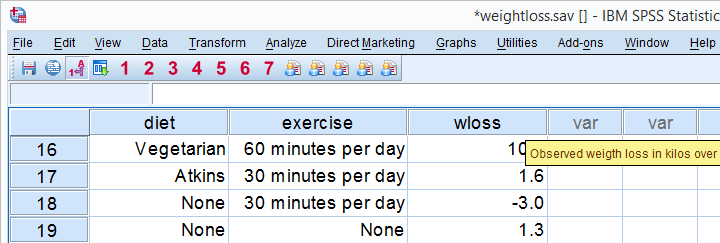## Research Question

How to lose weight effectively? Do diets really work and what about exercise? In order to find out, 180 participants were assigned to one of 3 diets and one of 3 exercise levels. After two months, participants were asked how many kilos they had lost. These data -partly shown above- are in weightloss.sav.
We're going to test if the means for weight loss after two months are the same for diet, exercise level and each combination of a diet with an exercise level. That is, we'll compare more than two means so we end up with some kind of ANOVA.

## Case Count and Histogram

We always want to have a basic idea what our data look like before jumping into any analyses. We first want to confirm that we really do have 180 cases. Next, we'd like to inspect the frequency distribution for weight loss with a histogram. We'll do so by running the syntax below.

*Inspect how many cases we have.

show n.

*Inspect histogram for weight loss.

frequencies wloss
/format notable /*= don't create table because it's too large.
/histogram.

## Result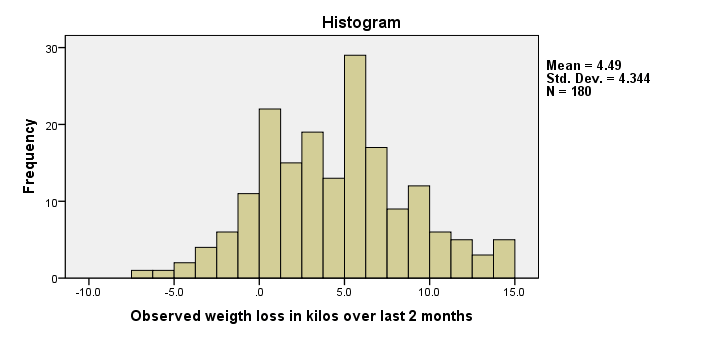We have 180 cases indeed. Importantly, the histogram of weight loss looks plausible. We don't see any very high or low values that we should set as user missing values. One or two participants gained some 7 kilos (weight loss = -7) and some managed to lose up to 15 kilos. Furthermore, weight loss looks reasonably normally distributed.

## Contingency Table Diet by Exercise

We now like to know how participants are distributed over diet and exercise. For our ANOVA, later on, we need to know if our design is balanced: are the percentages of participants in each diet equal over exercise levels? Some of you may notice that this question is actually the null hypothesis in a chi-square test. And that's exactly what we'll run next.

*See how participants are distributed over diet * exercise groups.

crosstabs diet by exercise
/statistics chisq.

## ResultNote that each cell (combination of diet and exercise level) holds 20 participants. Note that our chi-square value is 0 (not shown in screenshot). This implies that we're dealing with a balanced design, which is a good thing because unbalanced designs somewhat complicate a two-way ANOVA.

## Means Table

So did the diet and exercise have any effect? A very simple way for getting an idea of this is running a basic MEANS table.

*Inspect means for diet, exercise and diet by exercise.

means wloss by diet by exercise.

## Result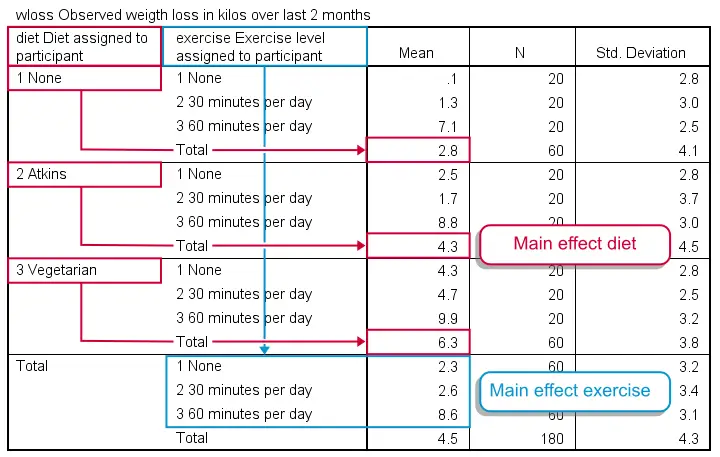It may take a minute to see the pattern in this table but I did my best to highlight it with colors. Note that participants without any diet -all exercise levels taken together- lost an average of 2.8 kilos. The Atkins and vegetarian diets resulted in 6.3 and 4.3 kilos of weight loss on average. This is the main effect for diet: the differences in weight loss attributable to diet while taking together all exercise levels. In a similar vein, we see a somewhat stronger main effect for exercise with means running from 2.3 up to 8.6 kilos.
An interesting question is whether the effect of exercise depends on the diet followed. This is what we call an interaction effect. We'll explain it in a minute by visualizing our means in a chart.

## Two Way ANOVA - Basic Idea

We just saw that different diets and exercise levels show different mean weight losses. However, we're looking at just a tiny sample. The situation in the (much larger) population may be different. Is it credible that we find these differences if neither diet nor exercise has any effect whatsoever in our population? We'll answer this question by running a two way ANOVA.

## ANOVA Assumptions

In short, the main statistical assumptions required for ANOVA are

• independent observations: this often means that each case (row of data values) must represent a separate person (or other “object”). It's not allowed for a single person to appear as more than one case, which holds for our data.
• homoscedasticity: the standard deviation of our dependent variable (weight loss) must be equal for each (diet/exercise) group of respondents. Our previous means table shows that they are pretty similar indeed. Nevertheless, we'll also test this assumption more formally with Levene's test which is included in SPSS ANOVA procedure.
• a normally distributed dependent variable in the population. Our previous histogram suggests this holds for our data. On top of that, the normality assumption is of minor importance for larger sample sizes due to the central limit theorem.

## SPSS Two Way ANOVA Menu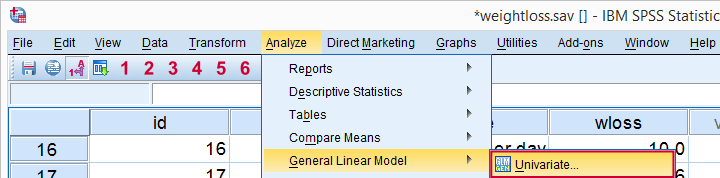We choose Univariate whenever we analyze just one dependent variable (weight loss), regardless how many independent variables (diet and exercise) we may have.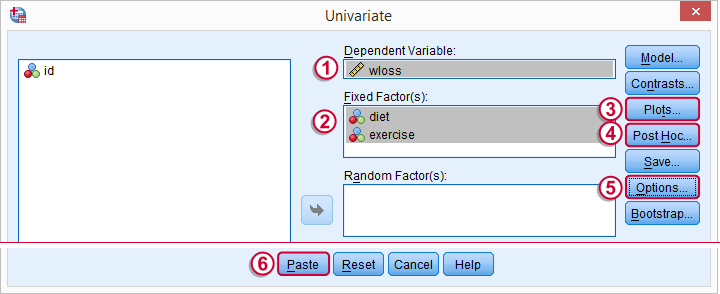Before pasting the syntax, we'll quickly jump into the subdialogs,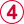andfor adjusting some settings.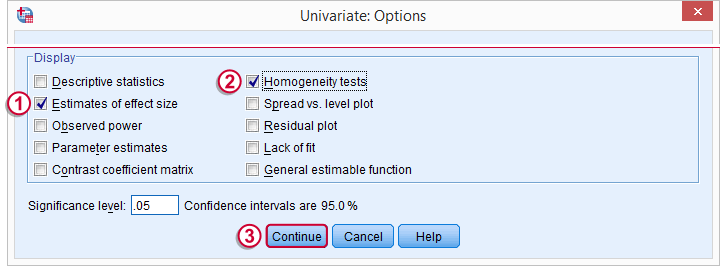Estimates of effect size will add partial eta squared in our output.Homogeneity tests refers to Levene’s test. It assesses whether the population variances of our dependent variable are equal over the levels of our factors. This assumption is required for ANOVA.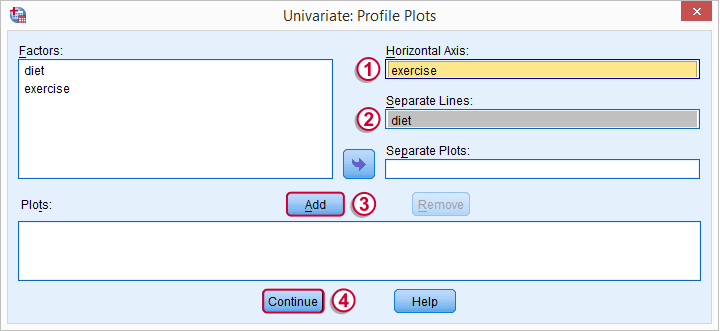Profile plots visualize means for each combination of factors. As we'll see in a minute, this gives a lot of insight into how our factors relate to our dependent variable and -possibly- interact while doing so.A basic ANOVA only tests the null hypothesis that all means are equal. If this is unlikely, then we'll usually want to know exactly which means are not equal. The most common post hoc test for finding out is Tukey’s HSD (short for Honestly Significant Difference).

## SPSS Two Way ANOVA Syntax

Following through all steps results in the syntax below. We'll run it and discuss the results.

*ANOVA with means plots, Tukey's post hoc test, Levene's test and Partial Eta Squared.

UNIANOVA wloss BY diet exercise
/METHOD=SSTYPE(3)
/INTERCEPT=INCLUDE
/POSTHOC=diet exercise (TUKEY)
/PLOT=PROFILE(exercise*diet)
/PRINT=ETASQ HOMOGENEITY
/CRITERIA=ALPHA(.05)
/DESIGN=diet exercise diet*exercise.

## Two Way ANOVA Output - Levene’s Test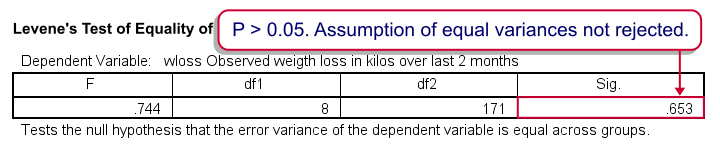Levene’s test does not reject the assumption of equal variances that's needed for our ANOVA results later on. We're good to go. Let's scroll down to the end of our output now for our profile plots first.

## Two Way ANOVA Output - Profile Plots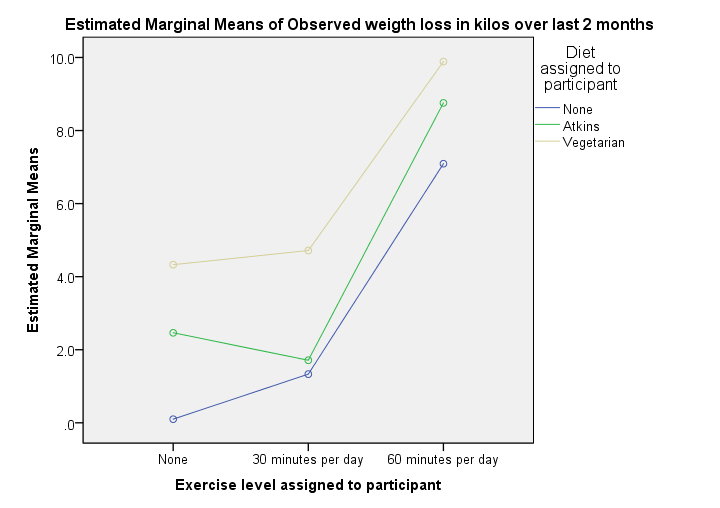This basically says it all. We see each line rise steeply between 30 to 60 minutes of exercise per day. Second, a vegetarian diet always resulted in more weight loss than the other diets. Both diet and exercise seem to have a main effect on weight loss.
So what about our interaction effect? Well, the effect of exercise is visualized as a line for each diet group separately. Since these lines look pretty similar, our plot doesn't show much of an interaction effect. However, we'll try to confirm this with a more formal test in a minute.
Technical note: the “estimated marginal means” are equal to the observed means in our previous means table because we tested the saturated model (consisting of all main and interaction effects as this is the default setting in UNIANOVA).

## Two Way ANOVA Output - Between Subjects Effects

Our means plot was very useful for describing the pattern of means resulting from diet and exercise in our sample. But perhaps things are different in the larger population. If neither diet nor exercise affect weight loss, could we find these sample results by mere sampling fluctuation? Short answer: no.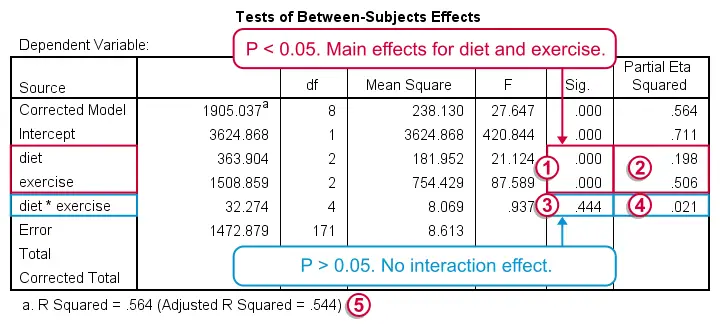In Tests of Between-Subjects Effects, we're interested in 3 rows: our 2 main effects (diet and exercise) and 1 interaction effect (diet * exercise). We usually ignore the other rows such as “Corrected Model” and “Intercept”.First the interaction: if the effect of exercise is the same for all diets, then there's a 0.44 probability (p-value under “Sig” for “significance”) of finding our sample results. We usually report our df (“degrees of freedom”), F-value and p-value for each of our 3 effects separately: “An interaction between diet and exercise could not be demonstrated, F(4,171) = .94, p = 0.44.”Further note that partial eta squared is only 0.021 for our interaction effect. This is basically negligible.If and only if there's no interaction effect, we'll look into the main effects, both of which have p = 0.000: if there's no main effects in our larger population, the probability of finding these sample main effects is basically zero.Partial eta squared is 0.51 for exercise and 0.20 for diet. That is, the relative impact of exerice is more than twice as strong as diet.Last but not least, adjusted r squared tells us that 54.4% of the variance in weight loss is attributable to diet and exercise. In social sciences research, this is a high value, indicating strong relationships between our factors and weight loss.

## Two Way ANOVA Output - Multiple Comparisons

We now know that the average weight loss is not equal for all different diets and exercise levels. So precisely which means are different? We can figure that out with post hoc tests, the most common of which is Tukey’s HSD, the output of which is shown below.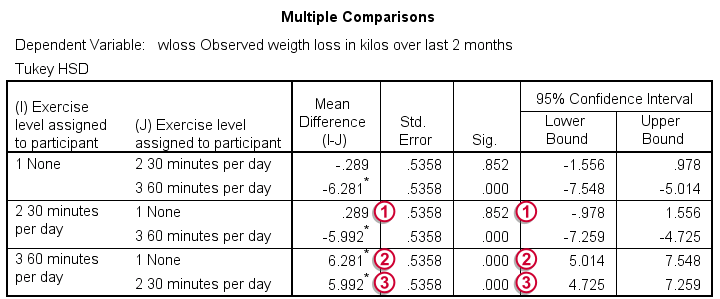For 3 means, 3 comparisons are made (a-b, b-c and a-c). Each is reported twice in this table, resulting in 6 rows.The difference in weight loss between no exercise and 30 minutes is 0.29 kilos. If it is zero in our larger populations, there's an 85.2% probability of finding this in our sample. Our results don't demonstrate any effect of 30 minutes of exercise as compared to no exercise.The difference between no exercise and 60 minutes is a whopping 6.28 kilos. Both the asterisk (*), confidence interval and p-value show that the difference is statistically significant.
A similar table for diet appears in the output but we'll leave it as an exercise to the reader to interpret it.

So that's about it. I hope you were able to follow the lines of thought in this tutorial and that they make some sense to you.

# Tell us what you think!

*Required field. Your comment will show up after approval from a moderator.

# THIS TUTORIAL HAS 28 COMMENTS:

•### By Woody on October 1st, 2020

Hi Geert

Thanks for writing. Yes I was thinking about the actual table setup.

It’s a very simple thing, but as a newcomer to SPSS, the actual setting up of table (step zero) was unclear. E.g. in Laerd, it described both the simplest steps (setting up table) through the complex (how to deal with two way ANOVA when variances are very different, as in my case).

Once I learned how to set up the table, your site for very helpful (compared to Laerd, e.g., which was fine, but excessively dense).

Best

Woody

•### By Ruben Geert van den Berg on October 1st, 2020

Hi Woody!

Thanks, perhaps we'll add it as a separate tutorial or include it if we ever find the time to update this tutorial.

Note that difference variances are only problematic in unbalanced designs. The usual way to go in that case is selecting "robust estimates" which result in the Welch statistic which is robust to violations of the homogeneity assumption. Some even argue you should always use the Welch statistic -perhaps true but somewhat unusual.

Kind regards!

Ruben

•### By Mark S on April 10th, 2021

I thought one should only conduct post-hoc analysis if the interaction effect is significant, which it's not in the shown example. If there isn't a sig interaction effect, then that means there aren't statistically significant differences across the means of individual groups (cells), right?

•### By Ruben Geert van den Berg on April 11th, 2021

Hi Mark, thanks for your question!

You're almost right:

-if the interaction effect is "significant", then we don't interpret any main effects. Instead, we'll look into simple effects as explained in Two-Way ANOVA with Interaction Tutorial.

-if the interaction effect is not "significant", then we interpret main effects (this tutorial).

-if a main effect is "significant", then we may look into post hoc tests (this tutorial and also SPSS ANOVA - Levene’s Test “Significant”).

Btw, some analysts also argue you should always/never run post hoc tests, regardless the main effect(s).

Hope that helps!

SPSS tutorials

•### By Matheus Fernandes Viola on September 15th, 2021

Hi, could you help me with a question?

I applied 4 doses of a drug (placebo, 5mg, 10mg and 15mg). Each dose was injected day and night (injection period). My dependent variable was neutrophil/lymphocyte ratio. I did not find a significant dose*injection period interaction, but the main effect of dose and injection period were significant. The post-hoc result indicates that all doses resulted in an increase in the neutrophil/lymphocyte ratio compared to the placebo dose and that animals injected at night had a greater increase in the neutrophil/lymphocyte ratio. The doubts here are:

1 - Ok, regardless of the dose used, there is an increase in relation to the placebo group. And this increase is greater when injected at night.

2 - Ok, but the placebo should not result in an increase in the neutrophil/lymphocyte ratio, so this difference between daytime and nighttime comes from the other doses (5mg, 10mg and 15mg)

3 - What is the correct way to know if this increase in the neutrophil/lymphocyte ratio was different or equal for day and night treatments that used equivalent doses?